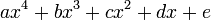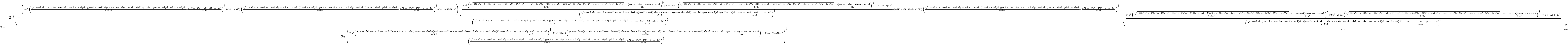# 07-401/More math-like

The first solution for$ax^4 + bx^3 + cx^2 + dx + e$, as presented by Maxima (use "tex(solve(a*x^4 + b*x^3 + c*x^2 + d*x + e,x))" and plug into the wiki)$x=-{{2^ {- {{3}\over{2}} }\,\sqrt{-{{\left(18\,a^2\,\left({{\sqrt{-256 \,a^3\,e^3-\left(-192\,a^2\,b\,d-128\,a^2\,c^2+144\,a\,b^2\,c-27\,b^4 \right)\,e^2-\left(\left(144\,a^2\,c-6\,a\,b^2\right)\,d^2+\left(18 \,b^3\,c-80\,a\,b\,c^2\right)\,d+16\,a\,c^4-4\,b^2\,c^3\right)\,e+27 \,a^2\,d^4-\left(18\,a\,b\,c-4\,b^3\right)\,d^3-\left(b^2\,c^2-4\,a \,c^3\right)\,d^2}}\over{6\,\sqrt{3}\,a^3}}-{{a\,\left(72\,c\,e-27\, d^2\right)-27\,b^2\,e+9\,b\,c\,d-2\,c^3}\over{54\,a^3}}\right)^{{{2 }\over{3}}}+\left(24\,a\,c-9\,b^2\right)\,\left({{\sqrt{-256\,a^3\,e ^3-\left(-192\,a^2\,b\,d-128\,a^2\,c^2+144\,a\,b^2\,c-27\,b^4\right) \,e^2-\left(\left(144\,a^2\,c-6\,a\,b^2\right)\,d^2+\left(18\,b^3\,c -80\,a\,b\,c^2\right)\,d+16\,a\,c^4-4\,b^2\,c^3\right)\,e+27\,a^2\,d ^4-\left(18\,a\,b\,c-4\,b^3\right)\,d^3-\left(b^2\,c^2-4\,a\,c^3 \right)\,d^2}}\over{6\,\sqrt{3}\,a^3}}-{{a\,\left(72\,c\,e-27\,d^2 \right)-27\,b^2\,e+9\,b\,c\,d-2\,c^3}\over{54\,a^3}}\right)^{{{1 }\over{3}}}+24\,a\,e-6\,b\,d+2\,c^2\right)\,\sqrt{{{36\,a^2\,\left( {{\sqrt{-256\,a^3\,e^3-\left(-192\,a^2\,b\,d-128\,a^2\,c^2+144\,a\,b ^2\,c-27\,b^4\right)\,e^2-\left(\left(144\,a^2\,c-6\,a\,b^2\right)\, d^2+\left(18\,b^3\,c-80\,a\,b\,c^2\right)\,d+16\,a\,c^4-4\,b^2\,c^3 \right)\,e+27\,a^2\,d^4-\left(18\,a\,b\,c-4\,b^3\right)\,d^3-\left(b ^2\,c^2-4\,a\,c^3\right)\,d^2}}\over{6\,\sqrt{3}\,a^3}}-{{a\,\left(72 \,c\,e-27\,d^2\right)-27\,b^2\,e+9\,b\,c\,d-2\,c^3}\over{54\,a^3}} \right)^{{{2}\over{3}}}+\left(9\,b^2-24\,a\,c\right)\,\left({{\sqrt{ -256\,a^3\,e^3-\left(-192\,a^2\,b\,d-128\,a^2\,c^2+144\,a\,b^2\,c-27 \,b^4\right)\,e^2-\left(\left(144\,a^2\,c-6\,a\,b^2\right)\,d^2+ \left(18\,b^3\,c-80\,a\,b\,c^2\right)\,d+16\,a\,c^4-4\,b^2\,c^3 \right)\,e+27\,a^2\,d^4-\left(18\,a\,b\,c-4\,b^3\right)\,d^3-\left(b ^2\,c^2-4\,a\,c^3\right)\,d^2}}\over{6\,\sqrt{3}\,a^3}}-{{a\,\left(72 \,c\,e-27\,d^2\right)-27\,b^2\,e+9\,b\,c\,d-2\,c^3}\over{54\,a^3}} \right)^{{{1}\over{3}}}+48\,a\,e-12\,b\,d+4\,c^2}\over{\left({{ \sqrt{-256\,a^3\,e^3-\left(-192\,a^2\,b\,d-128\,a^2\,c^2+144\,a\,b^2 \,c-27\,b^4\right)\,e^2-\left(\left(144\,a^2\,c-6\,a\,b^2\right)\,d^2 +\left(18\,b^3\,c-80\,a\,b\,c^2\right)\,d+16\,a\,c^4-4\,b^2\,c^3 \right)\,e+27\,a^2\,d^4-\left(18\,a\,b\,c-4\,b^3\right)\,d^3-\left(b ^2\,c^2-4\,a\,c^3\right)\,d^2}}\over{6\,\sqrt{3}\,a^3}}-{{a\,\left(72 \,c\,e-27\,d^2\right)-27\,b^2\,e+9\,b\,c\,d-2\,c^3}\over{54\,a^3}} \right)^{{{1}\over{3}}}}}}+\left(-216\,a^2\,d+108\,a\,b\,c-27\,b^3 \right)\,\left({{\sqrt{-256\,a^3\,e^3-\left(-192\,a^2\,b\,d-128\,a^2 \,c^2+144\,a\,b^2\,c-27\,b^4\right)\,e^2-\left(\left(144\,a^2\,c-6\, a\,b^2\right)\,d^2+\left(18\,b^3\,c-80\,a\,b\,c^2\right)\,d+16\,a\,c ^4-4\,b^2\,c^3\right)\,e+27\,a^2\,d^4-\left(18\,a\,b\,c-4\,b^3 \right)\,d^3-\left(b^2\,c^2-4\,a\,c^3\right)\,d^2}}\over{6\,\sqrt{3} \,a^3}}-{{a\,\left(72\,c\,e-27\,d^2\right)-27\,b^2\,e+9\,b\,c\,d-2\, c^3}\over{54\,a^3}}\right)^{{{1}\over{3}}}}\over{\left({{\sqrt{-256 \,a^3\,e^3-\left(-192\,a^2\,b\,d-128\,a^2\,c^2+144\,a\,b^2\,c-27\,b^4 \right)\,e^2-\left(\left(144\,a^2\,c-6\,a\,b^2\right)\,d^2+\left(18 \,b^3\,c-80\,a\,b\,c^2\right)\,d+16\,a\,c^4-4\,b^2\,c^3\right)\,e+27 \,a^2\,d^4-\left(18\,a\,b\,c-4\,b^3\right)\,d^3-\left(b^2\,c^2-4\,a \,c^3\right)\,d^2}}\over{6\,\sqrt{3}\,a^3}}-{{a\,\left(72\,c\,e-27\, d^2\right)-27\,b^2\,e+9\,b\,c\,d-2\,c^3}\over{54\,a^3}}\right)^{{{1 }\over{3}}}}}}}\over{3\,a\,\left({{36\,a^2\,\left({{\sqrt{-256\,a^3 \,e^3-\left(-192\,a^2\,b\,d-128\,a^2\,c^2+144\,a\,b^2\,c-27\,b^4 \right)\,e^2-\left(\left(144\,a^2\,c-6\,a\,b^2\right)\,d^2+\left(18 \,b^3\,c-80\,a\,b\,c^2\right)\,d+16\,a\,c^4-4\,b^2\,c^3\right)\,e+27 \,a^2\,d^4-\left(18\,a\,b\,c-4\,b^3\right)\,d^3-\left(b^2\,c^2-4\,a \,c^3\right)\,d^2}}\over{6\,\sqrt{3}\,a^3}}-{{a\,\left(72\,c\,e-27\, d^2\right)-27\,b^2\,e+9\,b\,c\,d-2\,c^3}\over{54\,a^3}}\right)^{{{2 }\over{3}}}+\left(9\,b^2-24\,a\,c\right)\,\left({{\sqrt{-256\,a^3\,e ^3-\left(-192\,a^2\,b\,d-128\,a^2\,c^2+144\,a\,b^2\,c-27\,b^4\right) \,e^2-\left(\left(144\,a^2\,c-6\,a\,b^2\right)\,d^2+\left(18\,b^3\,c -80\,a\,b\,c^2\right)\,d+16\,a\,c^4-4\,b^2\,c^3\right)\,e+27\,a^2\,d ^4-\left(18\,a\,b\,c-4\,b^3\right)\,d^3-\left(b^2\,c^2-4\,a\,c^3 \right)\,d^2}}\over{6\,\sqrt{3}\,a^3}}-{{a\,\left(72\,c\,e-27\,d^2 \right)-27\,b^2\,e+9\,b\,c\,d-2\,c^3}\over{54\,a^3}}\right)^{{{1 }\over{3}}}+48\,a\,e-12\,b\,d+4\,c^2}\over{\left({{\sqrt{-256\,a^3\, e^3-\left(-192\,a^2\,b\,d-128\,a^2\,c^2+144\,a\,b^2\,c-27\,b^4 \right)\,e^2-\left(\left(144\,a^2\,c-6\,a\,b^2\right)\,d^2+\left(18 \,b^3\,c-80\,a\,b\,c^2\right)\,d+16\,a\,c^4-4\,b^2\,c^3\right)\,e+27 \,a^2\,d^4-\left(18\,a\,b\,c-4\,b^3\right)\,d^3-\left(b^2\,c^2-4\,a \,c^3\right)\,d^2}}\over{6\,\sqrt{3}\,a^3}}-{{a\,\left(72\,c\,e-27\, d^2\right)-27\,b^2\,e+9\,b\,c\,d-2\,c^3}\over{54\,a^3}}\right)^{{{1 }\over{3}}}}}\right)^{{{1}\over{4}}}}}-{{\sqrt{{{36\,a^2\,\left({{ \sqrt{-256\,a^3\,e^3-\left(-192\,a^2\,b\,d-128\,a^2\,c^2+144\,a\,b^2 \,c-27\,b^4\right)\,e^2-\left(\left(144\,a^2\,c-6\,a\,b^2\right)\,d^2 +\left(18\,b^3\,c-80\,a\,b\,c^2\right)\,d+16\,a\,c^4-4\,b^2\,c^3 \right)\,e+27\,a^2\,d^4-\left(18\,a\,b\,c-4\,b^3\right)\,d^3-\left(b ^2\,c^2-4\,a\,c^3\right)\,d^2}}\over{6\,\sqrt{3}\,a^3}}-{{a\,\left(72 \,c\,e-27\,d^2\right)-27\,b^2\,e+9\,b\,c\,d-2\,c^3}\over{54\,a^3}} \right)^{{{2}\over{3}}}+\left(9\,b^2-24\,a\,c\right)\,\left({{\sqrt{ -256\,a^3\,e^3-\left(-192\,a^2\,b\,d-128\,a^2\,c^2+144\,a\,b^2\,c-27 \,b^4\right)\,e^2-\left(\left(144\,a^2\,c-6\,a\,b^2\right)\,d^2+ \left(18\,b^3\,c-80\,a\,b\,c^2\right)\,d+16\,a\,c^4-4\,b^2\,c^3 \right)\,e+27\,a^2\,d^4-\left(18\,a\,b\,c-4\,b^3\right)\,d^3-\left(b ^2\,c^2-4\,a\,c^3\right)\,d^2}}\over{6\,\sqrt{3}\,a^3}}-{{a\,\left(72 \,c\,e-27\,d^2\right)-27\,b^2\,e+9\,b\,c\,d-2\,c^3}\over{54\,a^3}} \right)^{{{1}\over{3}}}+48\,a\,e-12\,b\,d+4\,c^2}\over{\left({{ \sqrt{-256\,a^3\,e^3-\left(-192\,a^2\,b\,d-128\,a^2\,c^2+144\,a\,b^2 \,c-27\,b^4\right)\,e^2-\left(\left(144\,a^2\,c-6\,a\,b^2\right)\,d^2 +\left(18\,b^3\,c-80\,a\,b\,c^2\right)\,d+16\,a\,c^4-4\,b^2\,c^3 \right)\,e+27\,a^2\,d^4-\left(18\,a\,b\,c-4\,b^3\right)\,d^3-\left(b ^2\,c^2-4\,a\,c^3\right)\,d^2}}\over{6\,\sqrt{3}\,a^3}}-{{a\,\left(72 \,c\,e-27\,d^2\right)-27\,b^2\,e+9\,b\,c\,d-2\,c^3}\over{54\,a^3}} \right)^{{{1}\over{3}}}}}}}\over{12\,a}}-{{b}\over{4\,a}}$